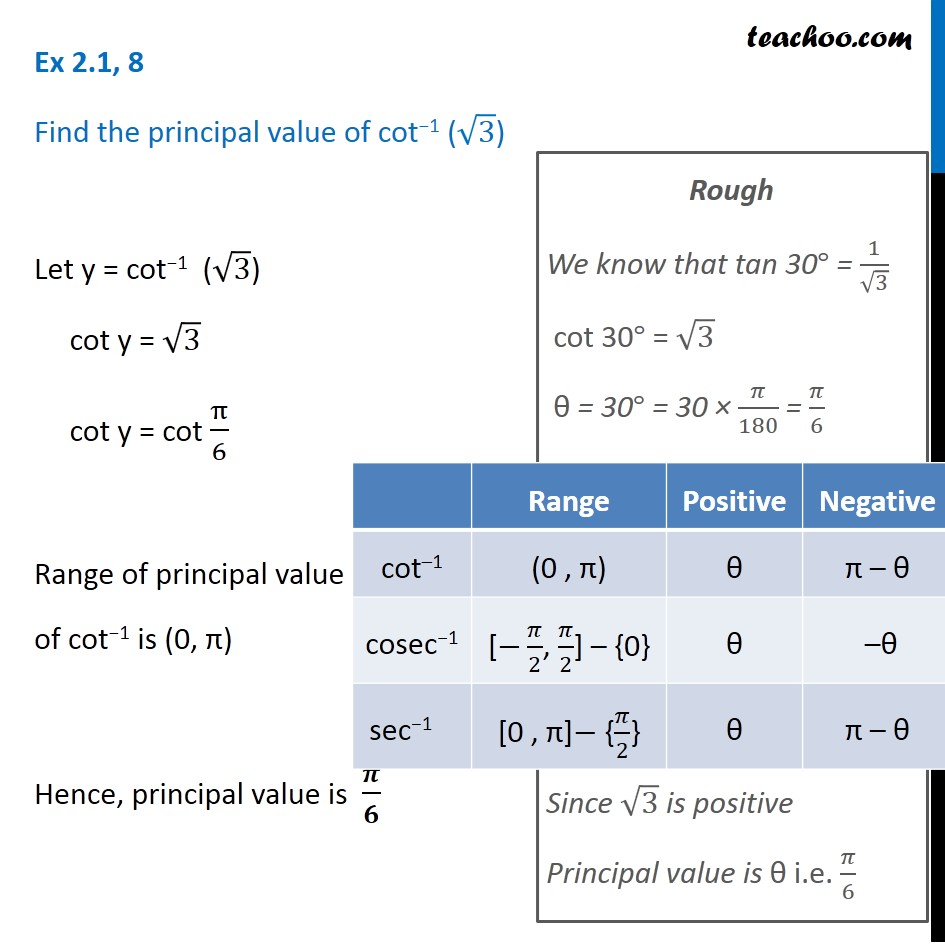Subscribe to our Youtube Channel - https://you.tube/teachoo

1. Chapter 2 Class 12 Inverse Trigonometric Functions
2. Serial order wise
3. Ex 2.1

Transcript

Ex 2.1, 8 Find the principal value of cot−1 (√3) Let y = cot−1 (√3) cot y = √3 cot y = cot π/6 Range of principal value of cot−1 is (0, π) Hence, principal value is 𝝅/𝟔 Rough We know that tan 30° = 1/√3 cot 30° = √3 θ = 30° = 30 × 𝜋/180 = 𝜋/6 Since √3 is positive Principal value is θ i.e. 𝜋/6

Ex 2.1